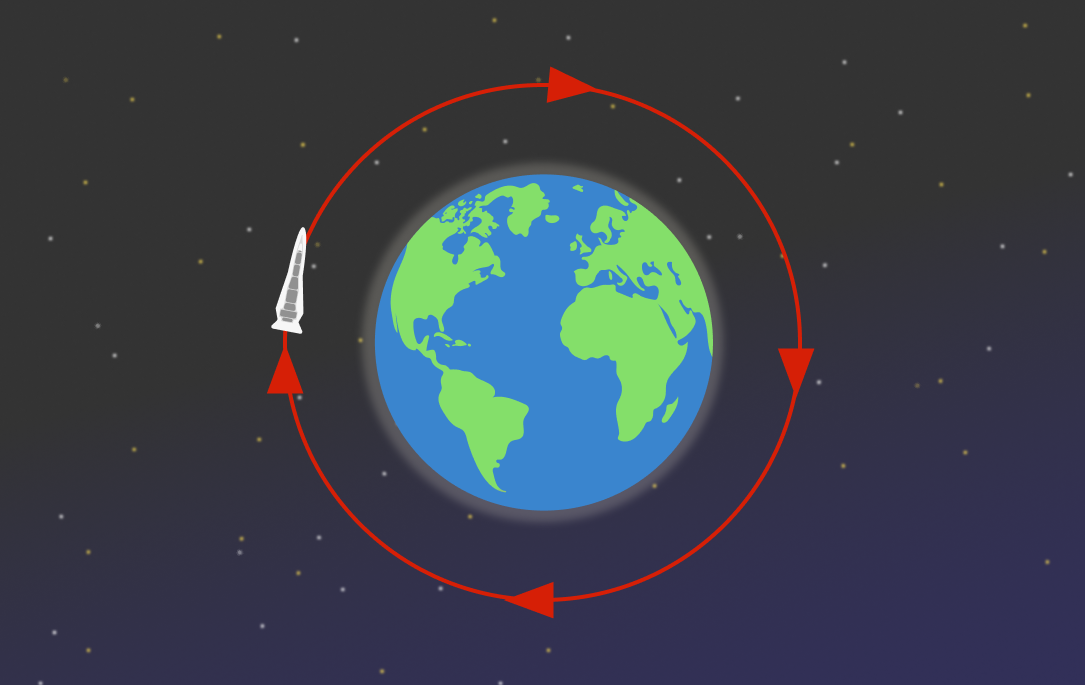# Spaceship Orbit

How fast $($in $\text{m/s})$ does a spaceship need to orbit around Earth to not spiral down or fly away from it?Details and Assumptions:

• The spaceship is $\SI{400}{\kilo\meter}$ above the surface of Earth.
• Earth's radius is $\SI{6371}{\kilo\meter}.$
• Gravitational acceleration on the spaceship is $\SI[per-mode=symbol]{8.69}{\meter\per\second\squared}$ towards the center of Earth.
×